FREE shipping to on qualifying orders when you spend or more. All prices ex. VAT.
We are currently open and operating as normal. Orders are being processed and dispatched on a daily basis.

Contact Angle: A Guide to Theory and Measurement

This guide will explain what a contact angle is and how it is measured. It will also show you how the Ossila Contact Angle Goniometer works and how to get the best measurement results. The guide is split into four sections:

What is a Contact Angle?

A contact angle gives us an indication of how well (or how poorly) a liquid will spread over a surface. While formulating an ink, contact angles provide a useful indicator of how a modification to the ink will affect its spreading.

A contact angle can be large or small, depending on the physical properties of the materials being investigated. Figure 1 shows three different droplets on a surface. The left-most droplet has a large contact angle, as it does not spread over the solid surface. The right-most droplet has a low contact angle, as it has spread well. This spreading is know as ‘wetting’, and a droplet either ‘wets’ or ‘dewets’ when deposited on a surface.

Figure 2 shows a 2D cross-section of a droplet on a solid surface. Locate the point at which the droplet outline intersects the solid surface. The angle between the droplet outline and the solid surface is the contact angle.

If we wanted a solution to spread more easily over a substrate, we could alter the solvents used in the formulation, and test them to check if this had increased its wetting capabilities. In this situation, a low contact angle would be the desired outcome.

Alternatively, we might be developing a waterproof coating for an item of clothing. In this case, a high contact angle would be desirable. We would alter the coating formulation, and use water droplets to determine which coating was more resistant to wetting.

Surface Tension

The surface tension of a droplet is determined by the interactions between its constituent molecules. The molecules in a droplet of liquid are shown in Figure 3. In the bulk of the droplet, intermolecular forces act upon a molecule from all sides equally. However, at the surface of the droplet, there is an absence of liquid molecules on the external side.

The molecules at the surface are more strongly bound to each other than the molecules in the bulk, as they are not being pulled from all sides. This means that it is more difficult for an object to penetrate the surface than it is for an object to move within the bulk once submerged.

Balanced Forces

There are three boundaries to consider when a droplet is in contact with a solid surface: the solid, the liquid, and the vapour (usually air) surrounding them.

Figure 4 shows a force diagram of the point at which a droplet edge meets a solid surface. The three arrows represent the forces exerted by the surface tensions at three interfaces: liquid-surface, liquid-vapour, and solid-vapour.

Each force is pulling away from the equilibrium point, so if the droplet is in equilibrium, then the forces are balanced and can be described by the following equation:Where cos θ gives the x-component of the liquid-vapour surface tension. This can be re-arranged to give: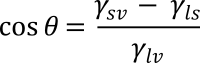This equation provides some useful information.

If γsv > γls, cos θ is positive, and θ is < 90o (and the droplet wets). This can occur with a high surface-energy solid (such as a metal), or a low surface-tension liquid.

If γsv < γls, then cos θ will be negative, and θ is therefore > 90o (and the droplet dewets). This can occur with a low surface-energy solid, or a high surface-tension liquid (such as water).

This may raise the question of how we equate surface energy density (units J/m2) with surface tension (units N/m).Surface energy density and surface tension can therefore be equated.

How is a Contact Angle Measured?

This section explains the basics of contact angle measurements and how they are usually performed. For specific details on how the Ossila Contact Angle Goniometer functions, please see the second and third sections of this guide.

Measurement Overview

The measurement involves two steps: Recording and Analysis. The equipment required to perform a contact angle measurement can be very simple. The most common method uses three basic components:

• A light source
• A camera
• A tilting stage
The measurement process is as follows:
1. The stage is flattened, so that the droplet does not move during deposition.
2. A droplet of liquid is deposited on to the stage.
3. The droplet is illuminated from behind, and an image is recorded by the camera.
4. The image is analysed using code or software, and a contact angle measurement is determined.

Recording an Image for Contact Angle Measurements

The first step in the measurement is to obtain an image of a droplet on a flat surface. There are several factors to consider when designing the optics of the measurement system. Optical components can significantly increase the cost of your setup, even if they are sometimes unnecessary.

Let us consider a photograph of a droplet on a substrate. What are the most important aspects of the image for the purposes of our measurement? Figure 1 shows some of the variables that can affect the quality of the droplet image. They are discussed in more detail below.

Image Size

Importantly, the image must contain the entire droplet; you can't measure the contact angle if you can't see the edge!

Clarity

You need to be able to see the droplet clearly. The better the clarity of the edges, the more accurately you will be able to measure the contact angle. A 'fuzzy' droplet edge will make it harder to see both the slope of the droplet and the place at which it meets the solid surface.

Clarity can be affected by factors such as camera resolution, image magnification, light levels, and contrast with the background.

Camera resolution

Camera resolution determines how many pixels represent the droplet edge. If an image is highly pixelated, it is hard to determine where the edge of the droplet is. For polynomial fitting (discussed later), the fewer the data-points, the less accurate the fit.

Magnification

Image magnification has an impact on resolution. The larger an optical image is, the more pixels it will cover and the higher resolution the image will be.

Contrast

Contrast is important for finding the droplet edge. If there is low contrast, then it will be hard to separate the droplet from its background. If you are using an edge-detection technique, contrast will determine detection accuracy.

Lighting

Light levels can also affect the measurement. You must be able to see the droplet to take a measurement. If the lighting is too light or too dark, the contrast of the droplet and background can be affected.

Depth of Field

You want the entire depth of the droplet to be in focus, otherwise it will be difficult to find the baseline through the blurry front of the droplet. This can be achieved by altering the ‘depth of field’ of the imaging system. The actual focal point of a lens is a specific distance from it. However, there is an area either side of the focal point which you consider to be in focus. This is known as the 'depth of field'. The depth of field can be altered by using an aperture. The image below shows the effect of an aperture on the light entering a camera. As the aperture is reduced in size, the light entering the camera is narrowed.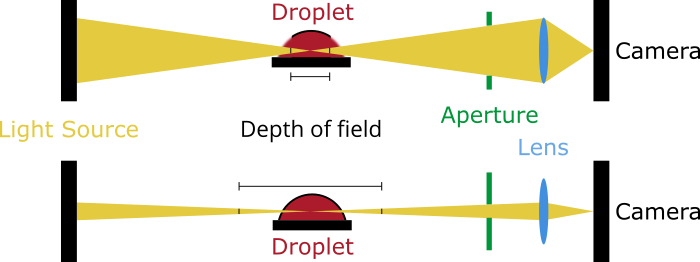Figure 2: The effect of an aperture on depth of field.

This results in two changes:
1. There is less overall light, so you need a bright background light.
2. The depth of field is increased and the ‘in focus’ region becomes longer.

As the aperture is narrowed, the size of the beam changes. If you consider the focal region to be within a certain beam width, you can extend this focal region away from the camera by narrowing the beam. The result of this is shown in Figure 2. In the first image, the depth of field starts and ends inside the droplet, so the front and back of the droplet are blurry. In the second image, the depth of field is wider than the whole droplet, so the entire droplet is within focus.

Analysis

Once an image has been obtained, most contact angle measurements follow three basic steps:

1. Define a baseline

The baseline of the measurement is the horizontal line that represents the solid on which the droplet is being deposited. The contact angle is the angle at the point where this baseline meets the droplet edge.

2. Trace the droplet edge

You can’t calculate the contact angle without knowing where the droplet edge meets the baseline. The droplet edge can be drawn by hand, detected programmatically, or estimated based on some assumptions about typical droplet shapes.

Next, you must determine the gradient of the tangent of the droplet edge at the point where it meets the baseline. As with the edge tracing, this can be done by hand with a protractor, or programmatically using software.

4. Calculate the contact angle

Once you have a baseline and a gradient, you can calculate the angle between them. To do this, you can use the trigonometric functions. For example, if the gradient is 3, then you can use the relationship tan(θ) = (opposite/adjacent) = (3/1) to calculate the angle. Once you have the baseline and the droplet edge, you can calculate the angle between them.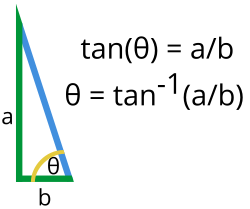Figure 4: Calculation of contact angle from the gradient of the slope where the droplet edge meets the baseline.

How Does the Ossila Contact Angle Goniometer Work?

In this guide, we will explain how the stages above are performed by the Ossila Contact Angle Goniometer, along with some tips and tricks to help you get the most out of your contact angle measurements.

1. Defining a baseline

In our Contact Angle Software, the baseline is defined by the user. Figure 5 shows a screenshot of the software measuring a droplet (b). The green lines in the image define boundaries of a region of interest (ROI), which is the part of the image that will be used for edge detection. The bottom boundary of this ROI is also the baseline of the measurement. There are sliders on each side of the display window (a), and their functions are as below:

• Slider on the right: Sets the baseline and the bottom boundary of the ROI.
• Slider at the top: Sets the left side of the ROI.
• Slider at the bottom: Sets the right side of the ROI.

You can move the sliders by dragging them, or by clicking the arrows for fine adjustment.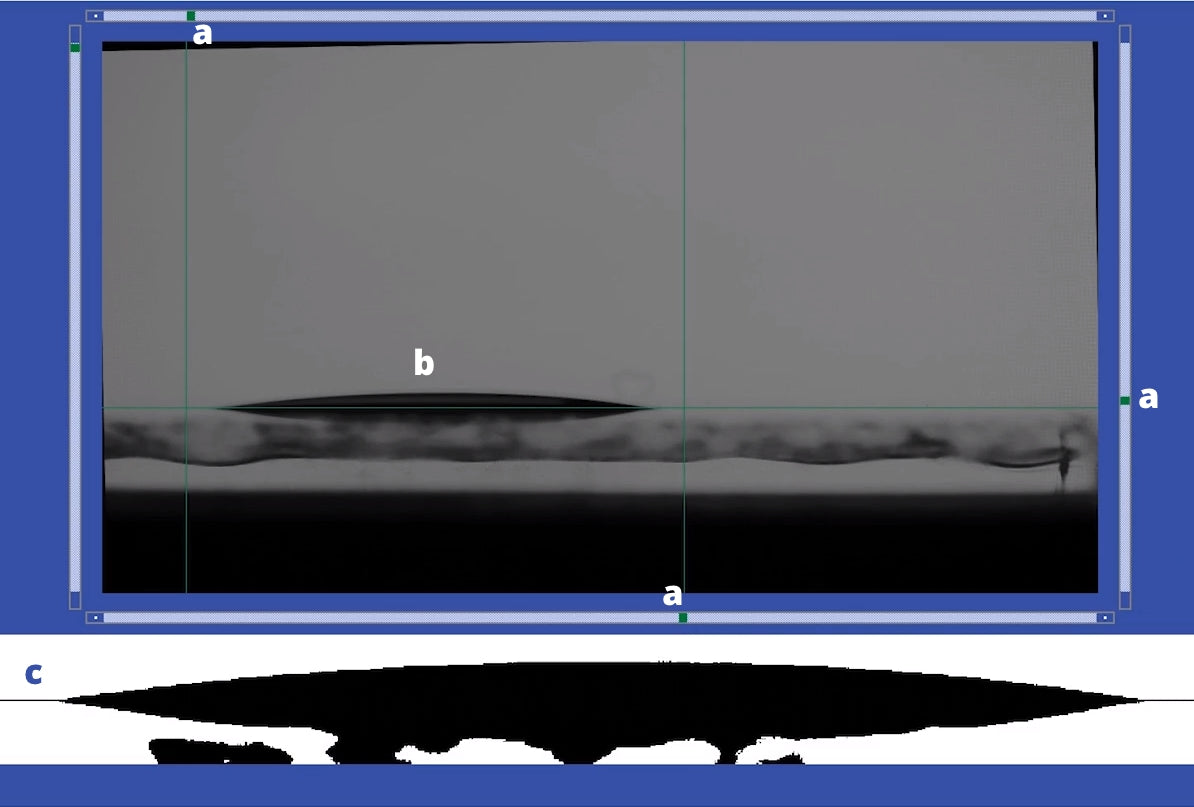Figure 5: Setting the region of interest in the Contact Angle Software showing a) region of interest sliders b) spreading droplet and c) region of interest.

The bottom part of the image (c) shows a magnified version of the ROI, which helps you with baseline positioning. The image in (c) is actually extended downwards (outside of the ROI) so that you can see if the baseline is in the correct vertical location. Only the area of (c) that is above the horizontal line is used for edge detection. By limiting the size of the ROI, you can remove any background objects that may affect detection of the droplet edge.

2. Tracing a droplet edge

First a threshold is applied to the area of the image within the region of interest. This threshold changes the image from grey-scale to pure black and white. The results of this are shown in Figure 5 (c). The software then searches each horizontal line and finds the coordinates of the first black pixel. This edge detection technique is shown in Figure 6. The green and blue circles indicate where the first black pixels are located in each line as the software searches from left to right (green) and then right to left (blue).

The technique described in section 2 provides a set of x,y coordinates of the droplet edge, as shown in Figure 7 (a). Using these coordinates, the Contact Angle software fits a second order polynomial to this edge (Figure 7 (b)).

Figure 7 (c) shows the gradient of the polynomial at the point where it crosses the baseline. The gradient of the polynomial can be calculated by taking its first derivative. You then input the x value at the point where the polynomial meets the baseline to determine the gradient at that point.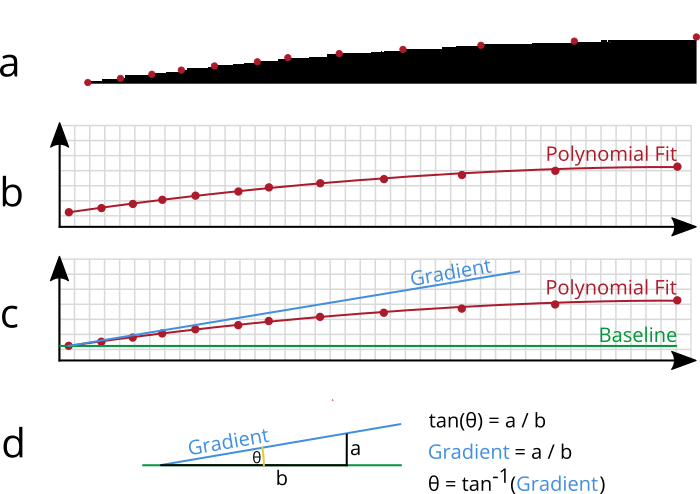Figure 7: a) Finding the edge of a droplet. b) Fitting a polynomial to the droplet edge. c) Finding the gradient of the polynomial. d) Calculating contact angle from the gradient.

4. Calculating the contact angle

Once we have the gradient of the line where it meets the baseline, we can use it to calculate the contact angle. Figure 7 (d) shows the components of the equation that was discussed in the previous section. The gradient at the baseline can be thought of as a triangle, with the gradient equal to the length of side a divided by the length of side b. Using the trigonometric relationships, we can determine the angle of the gradient. If the baseline is flat (which it always is), then the angle of the gradient is the same as the contact angle.

What Are the Tips, Tricks, and Limitations of the Measurement?

Setting a Baseline

Find the pointy bits.

The easiest way to set a baseline is to locate the point at which a droplet is mirrored vertically as it reflects off the substrate surface. Figure 8 shows an image of a droplet on a surface. The part of the droplet below the green line is actually a reflection. If you the baseline at the point where the real image meets its reflection, then you will likely be in the correct place.

As discussed in the very first section of this guide, a blurry droplet is difficult to measure. If you can't see the droplet well, then finding the baseline is more difficult. If the droplet is blurry, try screwing and unscrewing the camera lens to focus it, or try moving your substrate closer to and further from the camera - until it is in focus.

Don't go too low.

If the baseline is placed below the correct location, you will begin to detect the edge of the droplet reflection. Figure 9 shows how this will deform the polynomial fit of the droplet edge, with the edge of the reflected droplet shown by the blue circles. The gradient of the polynomial at the baseline will be negative, and the contact angle will be wrong. Therefore, it is better to set the baseline too high than too low.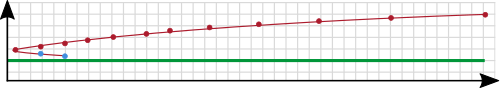Figure 9: Setting the baseline too low will effect polynomial fitting.
Flatten the stage.

Figure 10 shows the sample stage being tilted towards and away from the camera. Tilting the stage slightly towards the camera can help with baseline positioning, as the reflected droplet is useful for taking a measurement. If the stage is tilted too far towards the camera, the droplet will be viewed from above and the angle measurement will be less accurate. If the stage is tilted away from the camera, then some parts of the droplet will be obscured by the stage, making the measurement inaccurate.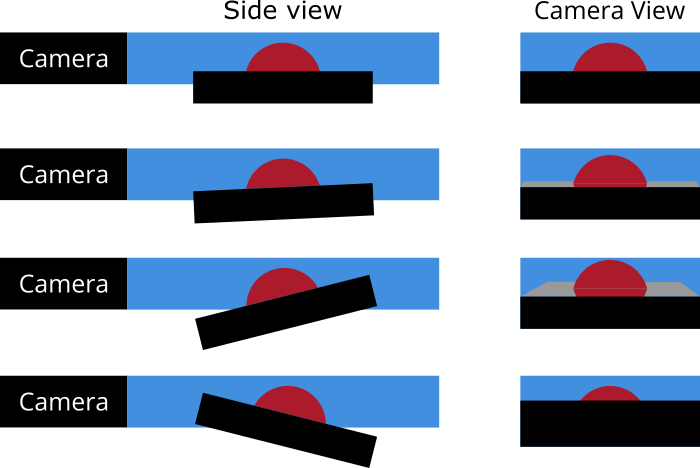Figure 10: Tilting the stage can help align the baseline, however tilting too far can alter the measurement.

Setting a Region of Interest

Make sure to include the whole droplet within the region of interest. Cropping the droplet edges will result in a wrong part of the droplet being measured. Also, make sure that there are no other objects or droplets within the region of interest, as these will be falsely detected as droplet edges

Taking a Black and White Threshold

Applying the black/white threshold to an image is most effective when there is a large contrast between the droplet and the background lighting. If a bright light is shone on the droplet from another direction, this can reduce the contrast - which will negatively affect the edge detection. It is therefore best to use the Contact Angle Goniometer in a shaded area, or in a room with low lighting.

Polynomial Fitting

A polynomial fit is only as good as the number of data points it can fit to. In the case of the contact angle measurement, the number of data points is the number of recorded pixels along the droplet edge. As discussed in the first section, this is a product of camera resolution, image magnification, and image clarity. When a droplet wets well to a surface, it will spread out very flatly. The flatter a droplet, the fewer pixels you can measure and the fewer data points you have for the polynomial fit. Therefore, when a droplet reaches a certain flatness, you cannot achieve an accurate measurement. For the Ossila Contact Angle Goniometer, the limit of measurement is 5°.